Updating search results...

# 40 Results

View
Selected filters:
• University of Alabama in Huntsville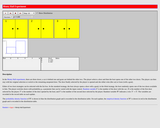Unrestricted Use
CC BY
Rating
0.0 stars

This resource consists of a Java applet and expository text. The applet is a simulation of the Monty Hall experiment: a car is behind one door, goats are behind the other two doors. The player chooses a door and then the host opens another door. The player is given the option of switching to the remaining door. The stochastic behavior of the host and the probability of the player switching can be specified.

Subject:
Mathematics
Statistics and Probability
Material Type:
Activity/Lab
Simulation
Provider:
University of Alabama in Huntsville
Provider Set:
Virtual Laboratories in Probability and Statistics
Author:
Kyle Siegrist
01/18/2006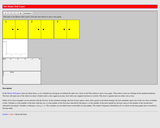Unrestricted Use
CC BY
Rating
0.0 stars

This resource consists of a Java applet and expository text. The applet consists of the Monty Hall game: a car is behind one door and goats are behind the other two doors. The player makes an initial choice and the host opens a different door. The player is then given the option of switching to the remaining door. The stochastic behavior of the host can be specified.

Subject:
Mathematics
Statistics and Probability
Material Type:
Activity/Lab
Interactive
Simulation
Provider:
University of Alabama in Huntsville
Provider Set:
Virtual Laboratories in Probability and Statistics
Author:
Kyle Siegrist
02/16/2011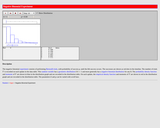Unrestricted Use
CC BY
Rating
0.0 stars

This resource consists of a Java applet and descriptive text. The applet illustrates the number of trials needed to get a specified number of successes in Bernoulli trials, in terms of random points on a discrete timeline. The applet illustrates the negative binomial distribution. The number of successes and the probability of success can be varied.

Subject:
Mathematics
Statistics and Probability
Material Type:
Activity/Lab
Interactive
Simulation
Provider:
University of Alabama in Huntsville
Provider Set:
Virtual Laboratories in Probability and Statistics
Author:
Kyle Siegrist
02/16/2011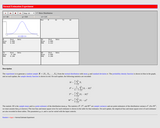Unrestricted Use
CC BY
Rating
0.0 stars

This resource consists of a Java applet and expository text. The applet simulates a random sample from a normal distribution, and computes standard point estimates of the distribution mean and standard deviation. The bias and mean square error are also computed.

Subject:
Mathematics
Statistics and Probability
Material Type:
Activity/Lab
Interactive
Simulation
Provider:
University of Alabama in Huntsville
Provider Set:
Virtual Laboratories in Probability and Statistics
Author:
Kyle Siegrist
02/16/2011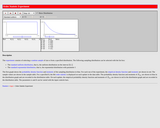Unrestricted Use
CC BY
Rating
0.0 stars

This resource consists of a Java applet and expository text. The applet simulates the order statistics of a random sample from a given distribution. The sample size, order, and sampling distribution can be specified.

Subject:
Mathematics
Statistics and Probability
Material Type:
Activity/Lab
Interactive
Simulation
Provider:
University of Alabama in Huntsville
Provider Set:
Virtual Laboratories in Probability and Statistics
Author:
Kyle Siegrist
02/16/2011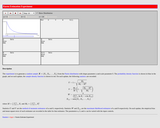Unrestricted Use
CC BY
Rating
0.0 stars

This resource consists of a Java applet and expository text. The applet simulates a random sample from a Pareto distribution, and computes standard point estimates of the shape parameter. The bias and mean square error are also computed.

Subject:
Mathematics
Statistics and Probability
Material Type:
Activity/Lab
Interactive
Simulation
Provider:
University of Alabama in Huntsville
Provider Set:
Virtual Laboratories in Probability and Statistics
Author:
Kyle Siegrist
02/16/2011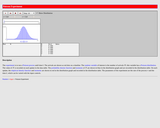Unrestricted Use
CC BY
Rating
0.0 stars

This resource consists of a Java applet and expository text. The applet simulates the arrivals in a Poisson process during a time interval. The length of the interval and the rate of the process can be varied. The applet illustrates the Poisson distribution and a special case of the central limit theorem.

Subject:
Mathematics
Statistics and Probability
Material Type:
Activity/Lab
Interactive
Simulation
Provider:
University of Alabama in Huntsville
Provider Set:
Virtual Laboratories in Probability and Statistics
Author:
Kyle Siegrist
02/16/2011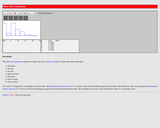Unrestricted Use
CC BY
Rating
0.0 stars

This resource consists of a Java applet and expository text. The experiment consists of rolling 5 fair dice. The random variable of interest is the type of hand.

Subject:
Mathematics
Statistics and Probability
Material Type:
Activity/Lab
Interactive
Simulation
Provider:
University of Alabama in Huntsville
Provider Set:
Virtual Laboratories in Probability and Statistics
Author:
Kyle Siegrist
02/16/2011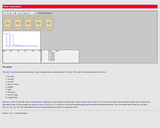Unrestricted Use
CC BY
Rating
0.0 stars

This resource consists of a Java applet and expository text. The applet simulates the basic poker experiment of dealing 5 cards from a standard deck. The random variable of interest is the type of hand.

Subject:
Mathematics
Statistics and Probability
Material Type:
Interactive
Provider:
University of Alabama in Huntsville
Provider Set:
Virtual Laboratories in Probability and Statistics
Author:
Kyle Siegrist
11/04/2014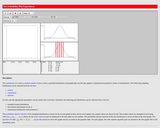Unrestricted Use
CC BY
Rating
0.0 stars

This resource consists of a Java applet and Expository text. The applet simulates the probability plot that compares the empirical quantiles of a sample from a sampling distribution to the distribution quantiles of a test distribution. The sampling distribution, test distribution, and sample size can be specified.

Subject:
Mathematics
Statistics and Probability
Material Type:
Activity/Lab
Interactive
Simulation
Provider:
University of Alabama in Huntsville
Provider Set:
Virtual Laboratories in Probability and Statistics
Author:
Kyle Siegrist
02/16/2011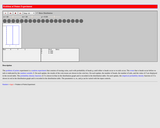Unrestricted Use
CC BY
Rating
0.0 stars

This resource consists of a Java applet and descriptive text. The applet illustrates the problem of points: a coin is tossed until either n heads occurs or m tails occurs. The parameters n, m, and the probability of heads can be varied.

Subject:
Mathematics
Statistics and Probability
Material Type:
Activity/Lab
Interactive
Simulation
Provider:
University of Alabama in Huntsville
Provider Set:
Virtual Laboratories in Probability and Statistics
Author:
Kyle Siegrist
02/16/2011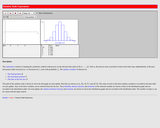Unrestricted Use
CC BY
Rating
0.0 stars

This resource consists of a Java applet and descriptive text. The applet illustrates a random walk on a discrete time interval from 0 to n. The time n and the probability of a step to the right can be varied. The random variables of interest are the final position, the maximum position, and the time of the last zero.

Subject:
Mathematics
Statistics and Probability
Material Type:
Activity/Lab
Interactive
Simulation
Provider:
University of Alabama in Huntsville
Provider Set:
Virtual Laboratories in Probability and Statistics
Author:
Kyle Siegrist
02/16/2011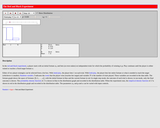Unrestricted Use
CC BY
Rating
0.0 stars

This resource consists of a Java applet and expository text. The applet simulates the red and black experiment in which a player bets on a sequence of Bernoulli trials until a target fortune is reached or the player is ruined. The initial fortune, target fortune, and trial win probability can be varied, and the user can select either of two basic strategies: timid play or bold play.

Subject:
Mathematics
Statistics and Probability
Material Type:
Interactive
Provider:
University of Alabama in Huntsville
Provider Set:
Virtual Laboratories in Probability and Statistics
Author:
Kyle Siegrist
11/04/2014Unrestricted Use
CC BY
Rating
0.0 stars

This resource consists of a Java applet and expository text. The applet allows the student to play the game of red and black, by betting on a sequence of Bernoulli trials until either a target is reached or the player is ruined. The target, initial fortune, and trial win probability can be varied.

Subject:
Mathematics
Statistics and Probability
Material Type:
Activity/Lab
Interactive
Simulation
Provider:
University of Alabama in Huntsville
Provider Set:
Virtual Laboratories in Probability and Statistics
Author:
Kyle Siegrist
02/16/2011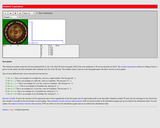Unrestricted Use
CC BY
Rating
0.0 stars

This resource consists of a Java applet and expository text. The applet is a simulation of the standard casino roulette game. The random variables of interest are the winning under various bets.

Subject:
Mathematics
Statistics and Probability
Material Type:
Activity/Lab
Interactive
Simulation
Provider:
University of Alabama in Huntsville
Provider Set:
Virtual Laboratories in Probability and Statistics
Author:
Kyle Siegrist
02/16/2011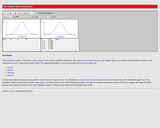Unrestricted Use
CC BY
Rating
0.0 stars

The resource consists of a Java applet and expository text. The applet illustrates the distribution of the sample mean of a random sample from a given distribution. The sample size and the sampling distribution can be specified. The applet illustrates the central limit theorem.

Subject:
Mathematics
Statistics and Probability
Material Type:
Activity/Lab
Interactive
Simulation
Provider:
University of Alabama in Huntsville
Provider Set:
Virtual Laboratories in Probability and Statistics
Author:
Kyle Siegrist
02/16/2011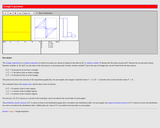Unrestricted Use
CC BY
Rating
0.0 stars

This resource consists of a Java applet and expository text. The applet illustrates the triangle experiment: A stick is broken randomly into three parts. The events of interest are that the pieces form an acute triangle, an obtuse triangle, or no triangle.

Subject:
Mathematics
Statistics and Probability
Material Type:
Activity/Lab
Interactive
Simulation
Provider:
University of Alabama in Huntsville
Provider Set:
Virtual Laboratories in Probability and Statistics
Author:
Kyle Siegrist
02/16/2011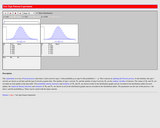Unrestricted Use
CC BY
Rating
0.0 stars

This resource consists of a Java applet and expository text. The applet illustrates the arrivals in a Poisson process where each arrival, independently, is one of two types. The time interval, the rate of the process, and the probability that an arrival is type 1 can be varied. The applet illustrates "splitting" a Poisson process.

Subject:
Mathematics
Statistics and Probability
Material Type:
Activity/Lab
Interactive
Simulation
Provider:
University of Alabama in Huntsville
Provider Set:
Virtual Laboratories in Probability and Statistics
Author:
Kyle Siegrist
02/16/2011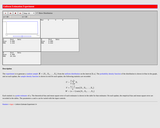Unrestricted Use
CC BY
Rating
0.0 stars

This resource consists of a Java applet and expository text. The applet simulates a random sample from the uniform distribution on the interval [0,a], and computes standard point estimates of a. The bias and mean square error are also computed.

Subject:
Mathematics
Statistics and Probability
Material Type:
Activity/Lab
Interactive
Simulation
Provider:
University of Alabama in Huntsville
Provider Set:
Virtual Laboratories in Probability and Statistics
Author:
Kyle Siegrist
02/16/2011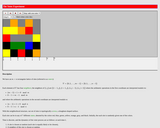Unrestricted Use
CC BY
Rating
0.0 stars

This resource consists of a Java applet and expository text. The applet is a simulation of the voter experiment, an interacting partile system that consists of a rectangular array of sites. At each discrete time unit, a site is chosen at random, a neighboring site is chosen, and the color of the first site is changed to that of the neighbor. The applet illustrates clustering of the colors and convergence to consensus.

Subject:
Mathematics
Statistics and Probability
Material Type:
Activity/Lab
Interactive
Simulation
Provider:
University of Alabama in Huntsville
Provider Set:
Virtual Laboratories in Probability and Statistics
Author:
Kyle Siegrist